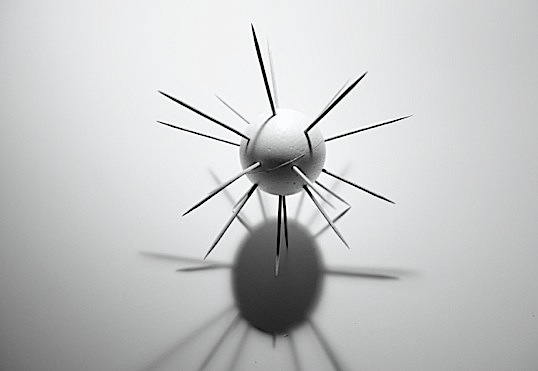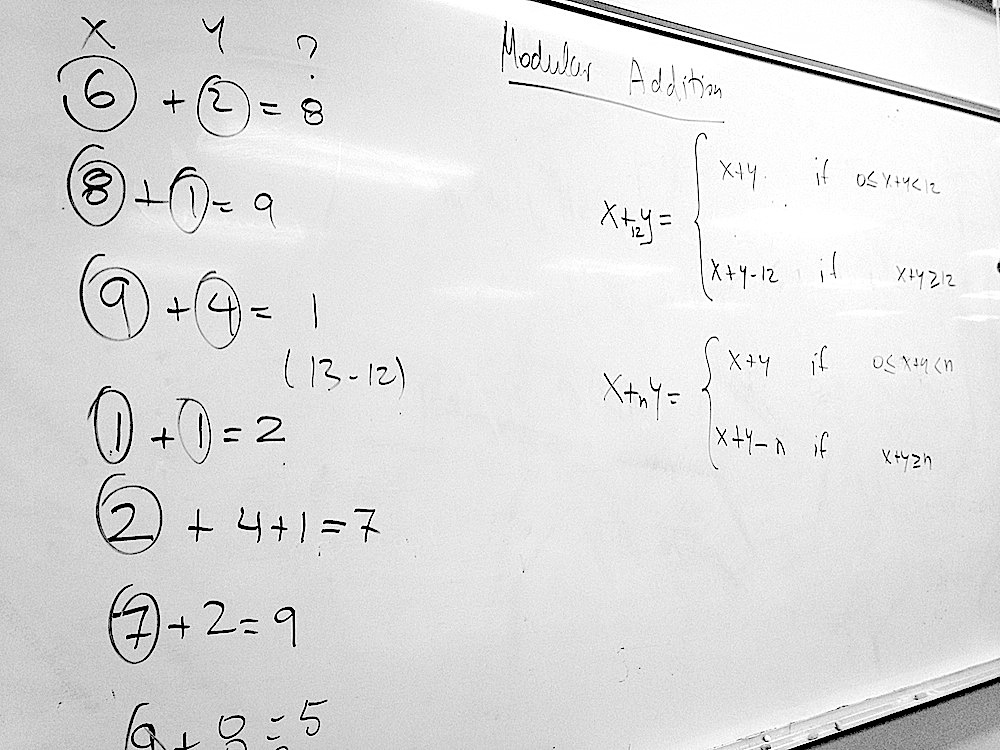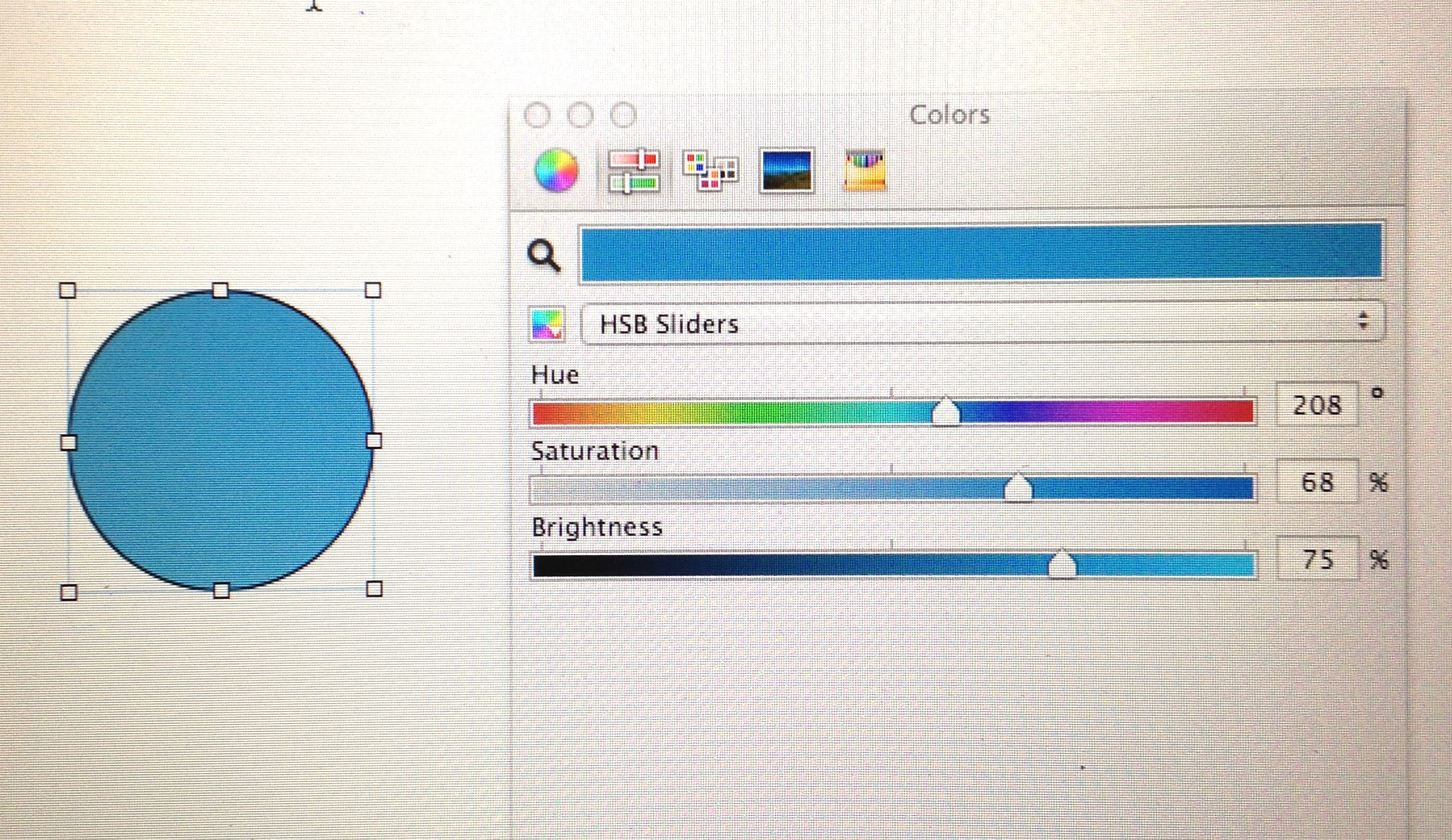## Discovering Group Theory, Day 7

On Day 7, we moved on to the symmetries of a square. Using the same methods as for the equilateral triangle, we got the structure of D4, though we left some derivations for a homework assignment. We then made an educated guess about the structure of the symmetry group of a pentagon, D5, and then generalized to the symmetries of an n-dimensional regular polygon, which led us to the formal definition of Dn.

## Discovering Group Theory, Day 6

On Day 6, we dove into dihedral group D3, which students have been exploring independently as part of their homework and in the context of the symmetries of an equilateral triangle.

• We tackled the group using three methods:
• physically manipulating a cutout of an equilateral triangle,
• drawing diagrams of various operations, simple (single rotation or reflection) and combined (rotation followed by a reflection, etc.), and
• working out the results of combined operations algebraically.This was an attempt to unify the physical experience of manipulating an object, visually representing the effects of those manipulations on the object, and expressing such operations symbolically.Symmetry transformations of an equilateral triangle.

• We also revisited the already familiar concepts of identity, inverse, generators, group order, and element order, and constructed the Cayley table for D3.
• We noticed that the order in which rotations and reflections are carried out does matter, which motivated the introduction of the commutativity as a property of two elements. This led us to the definitions of Abelian and a non-Abelian groups.

## Discovering Group Theory, Day 5

On Day 5, was devoted to the discussion of  “Mathematics for Human Flourishing,” a farewell address given by Francis Su at at the end of his term as the President of the Mathematical Association of America.

Students shared their own experiences with mathematics and how those experiences shaped their attitude toward the subject.An excerpt from Francis Su’s speech.

## Color Physics @Hampshire, Day 7

On Day 7, we finally got to understand what electromagnetic waves are.

• Students used a wonderful HTML-based simulation of a radiating charge to explore how motion of an electrically charged particle affects its electric field lines. They wrote their observations, the key ones which we discussed as a class.A screenshot of the PhET simulation of an oscillating electric charge and its electric field.
• After briefly talking about the creation of magnetic field by a changing electric field and vice versa (mostly in a hand-wavy way), we moved on to the more familiar representation of the electromagnetic wave far away from wiggling charged particle. For this we used a very nice Java simulation developed by Juan M. Aguirregabiria, the screenshot of which is shown below. The shift inspired a good discussion of the pros and cons of the two visual representations of the electric field (arrows and field lines) and the relation between the depictions of electromagnetic radiation in the two simulations.A screenshot of a simulation of a plane electromagnetic wave.

• After discussing different types of electromagnetic radiation (UV, visible light, WiFi, microwave) and its effects and uses, we began discussing what it takes to make a wave in general.

## Color Physics @Hampshire, Day 6

On Day 6, we began to unpack the statement that light is an electromagnetic wave, leaving the particle nature of light for a later discussion.A toy model of the electric field lines of a spherically symmetric electric charge made of styrofoam sphere and toothpicks.
• We discussed electric charge and electric force.
• We introduced the concept of electric field!
• We learned about two ways of visually representing the strength and the direction of the electric field, one with arrows at various points, the other with field lines. To get a better sense of the spatial distribution of the field, students built with styrofoam balls and toothpicks 3D models of the electric field of a spherically symmetric charge.
• We worked on conceptual problems testing students’ understanding of the above concepts.

## Color Physics @Hampshire, Day 5

On Day 5, we were familiarizing ourselves with notation and problem-solving strategies.

• We completed more examples of units conversions and scientific notation.
• We practiced problem-solving with examples relating speed, distance, and time

We ended the class by deflecting a stream of water with an electrically charged balloon as a segue to the discussion of light as an electromagnetic wave!

## Color Physics @Hampshire, Day 4

Day 4 consisted of wrapping up the discussion of HSB, introducing the RGB model, and setting the groundwork for the upcoming discussion of physics.

• Review of of Hue, Saturation, and Brightness
• HSB cylinder
• Additive color mixing: We organized our observations of mixing of colored light from the previous class and introduced the RGB color model.
• Primary colors in additive mixing
• Complementary colors in the additive system
• RBG color model
• RGB cube
• Measurement in physics:
• What is science?
• Units and unit conversions
• Scientific notation and orders of magnitude. We watched Powers of Ten to get a better sense of what different powers of ten mean in terms of different scales, so that we can assess our answers to numerical problems.Powers of Ten™

Other Resources: A student pointed me to an interactive catalog of objects of a vast range of sizes, organized by powers of ten http://htwins.net/scale2/

## Discovering Group Theory, Day 4

Realizing that there was much to be gained from using now familiar cyclic groups to introduce more group properties, we postponed the exploration of dihedral groups and instead spent the day exploring.

• The review of group properties
• After reviewing the structure of particular examples of cyclic groups (C1, C2, C3, C4, and C6) students extrapolated the properties of Cn, which led us to the formal definition of cyclic groups.
• Modular Addition and (Zn,+n)
• We built on our existing knowledge of how to calculate the hour reading of a clock to motivate modular addition. Students were asked to come up with a general mathematical formula which can be used to do the calculations.
• Working in pairs, students then investigated whether (Z4,+4) forms a group by checking if it satisfies the four axioms and constructing the Cayley table.  This exercises broadened our understanding of group “multiplication,” which was originally introduced as a way to represent compounding effects of subsequent rotations of a polygon. It became clear why we have been using quotes around the word multiplication.Realizing we already know how to do modular addition.
• After comparing C4 and Z4, we introduced the idea of a group isomorphism.
• What is Not a Group? Students wanted to know more about when a set and a “product” do not form a group, so we went through a couple of examples in which one or more of the four axioms is violated (especially associativity).

## Discovering Group Theory, Day 3

On Day 3, we did two different sets of activities:

1.  We revisited the definition of a group and its properties in the context of cyclic groups, namely C1, C2, C3, C4, and C6,  encoding the rotational symmetry of polygons we played with on Day 2. Particular features of the cyclic groups prompted a natural introduction to a few new concepts and new terminology:
• Order of a group
• Order of an element of a group
• Group generators
• Group multiplication (Cayley) table, which students worked out for C3, C4, and C6 .
2. We began exploring the symmetries of a rectangle, but this time including the reflections. This allowed us study the above-listed concepts in a different context and practice carrying out group multiplication and finding inverses.

The plan was to use 2 to segue into dihedral groups, but it seems more pedagogical to spend another class or two on exploring the cyclic groups in more depth.

## Color Physics @Hampshire, Day 3

Topics

• Saturation, hue, and brightness
• Additive Color Mixing

We started Day 3 by playing with the HSB sliders on a computer software to determine which properties of perceived color are referred to as hue, saturation, and brightness.Playing with Hue, Saturation, and Brightness sliders to find out how they affect the color

After using the hand-held spectrometers to investigate the spectrum of a computer screen, cellphone screens, and the video projector, students made predictions about how brightness, saturation, and hue may be affected by mixing different amounts of red, green, and blue light and tested their predictions using primary color light sticks.Making a prediction about color mixing and testing them.Mixing light with primary colors and investigating how digital images recored by a cellphone differ from what is seen by the naked eye.